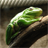# QlikView App Dev

Discussion Board for collaboration related to QlikView App Development.

Announcements
Action-Packed Learning Awaits! QlikWorld 2023. April 17 - 20 in Las Vegas: REGISTER NOW
cancel
Showing results for
Did you mean:Creator

## New Buyers to a Branch

Hi all I am wanting to count the number of new buyers to a specific branch.

New buyers would be a buyer who has bought a unit in June but not between Dec - May at that same branch.

 =aggr(if(

count(

{< SOLD.YrMthSeq= {"<=\$(vRptYrMthSeqTY ) >=\$(vRptYrMthSeqTY) "},

UNIT.STOCKSTATUS={'Sold'} >}

UNIT.TOTALSOLD) > 0

and

count(

{<SOLD.YrMthSeq= {"<=\$(=\$(vRptYrMthSeqTY) -1) >=\$(=\$(vRptYrMthSeqTY) -6)"},

UNIT.STOCKSTATUS={'Sold'} >}

UNIT.TOTALSOLD) = 0 ,

My dimension is branch and the first set analysis is asking if they have bought something in june the second is if they have bought something between Dec - May.

Any help would be very appreciated.

Thanks

Gareth

1 Solution

Accepted SolutionsPartner - Champion III

My bad - the Distinct should be before the inner Aggr():

=Sum(Aggr(

Count(DISTINCT Aggr(

If(Count({<SOLD.YrMthSeq={"<=\$(vRptYrMthSeqTY) >=\$(vRptYrMthSeqTY)"}, UNIT.STOCKSTATUS={'Sold'}>} UNIT.TOTALSOLD) > 0

And Count({<SOLD.YrMthSeq={"<=\$(=\$(vRptYrMthSeqTY)-1)>=\$(=\$(vRptYrMthSeqTY)-6)"}, UNIT.STOCKSTATUS={'Sold'} >} UNIT.TOTALSOLD) = 0,

,Branch))

Logic will get you from a to b. Imagination will take you everywhere. - A Einstein
12 RepliesSpecialist III

Hi Gareth,

I believe you also want to aggregate by Branch name or no as well. If that doesn't work, would you mind posting some sample data?

ThanksMVP

I would first check if these give you correct values when you use them in a straight table with BUYER.B_NO as Dimension:

Count({< SOLD.YrMthSeq= {"<=\$(vRptYrMthSeqTY ) >=\$(vRptYrMthSeqTY) "},UNIT.STOCKSTATUS={'Sold'} >} UNIT.TOTALSOLD)

Count({<SOLD.YrMthSeq= {"<=\$(=\$(vRptYrMthSeqTY) -1) >=\$(=\$(vRptYrMthSeqTY) -6)"}, UNIT.STOCKSTATUS={'Sold'} >} UNIT.TOTALSOLD)

If those are correct then we can make sure that we are moving in the right direction, but if not I would focus my attention of fixing them first.

HTH

Best,

SunnyPartner - Specialist

Hello Gareth,

Try to use sum(if ()) construction to achive require result.

sum(

 if(

count(

{< SOLD.YrMthSeq= {"<=\$(vRptYrMthSeqTY ) >=\$(vRptYrMthSeqTY) "},

UNIT.STOCKSTATUS={'Sold'} >}

UNIT.TOTALSOLD) > 0

and

count(

{<SOLD.YrMthSeq= {"<=\$(=\$(vRptYrMthSeqTY) -1) >=\$(=\$(vRptYrMthSeqTY) -6)"},

UNIT.STOCKSTATUS={'Sold'} >}

UNIT.TOTALSOLD) = 0 ,

1, 0)

)

Hope this helps you.

Regards,

AndreiCreator
Author

Hi Sunny,

Originally I had =if([June Buyers] >0 and [May - Dec Buyers] = 0 , 1) as the expression

with [June Buyers] being Count({< SOLD.YrMthSeq= {"<=\$(vRptYrMthSeqTY ) >=\$(vRptYrMthSeqTY) "},UNIT.STOCKSTATUS={'Sold'} >} UNIT.TOTALSOLD)

and

[May - Dec Buyers] being Count({<SOLD.YrMthSeq= {"<=\$(=\$(vRptYrMthSeqTY) -1) >=\$(=\$(vRptYrMthSeqTY) -6)"}, UNIT.STOCKSTATUS={'Sold'} >} UNIT.TOTALSOLD)

this worked and flagged the buyers that fall into this criteria.

But I need this to be totalled up for each Branch.

Thanks

GarethCreator
Author

Thanks Andrei but that is just showing null values?Partner - Specialist

Hm... very strange..

Does it work if you put your original flag into sum()- function?

 Gareth Cox-Thorpe wrote: Originally I had =if([June Buyers] >0 and [May - Dec Buyers] = 0 , 1) as the expression

AndreiPartner - Champion III

I think this is closer to what you need. Change the Branch field to the correct field name.

=Sum(Aggr(

Count(Aggr(

If(Count({<SOLD.YrMthSeq={"<=\$(vRptYrMthSeqTY) >=\$(vRptYrMthSeqTY)"}, UNIT.STOCKSTATUS={'Sold'}>} UNIT.TOTALSOLD) > 0

And Count({<SOLD.YrMthSeq={"<=\$(=\$(vRptYrMthSeqTY)-1)>=\$(=\$(vRptYrMthSeqTY)-6)"}, UNIT.STOCKSTATUS={'Sold'} >} UNIT.TOTALSOLD) = 0,

,Branch))

Logic will get you from a to b. Imagination will take you everywhere. - A EinsteinPartner - Champion III

Andrei

That expression does not work as you are trying to nest a Count() inside a Sum(). You need an Aggr() for the inner aggregation.

Logic will get you from a to b. Imagination will take you everywhere. - A EinsteinPartner - Specialist

Oh.. yes.. indeed 🙂

Thanks Jonathan!Community Browser